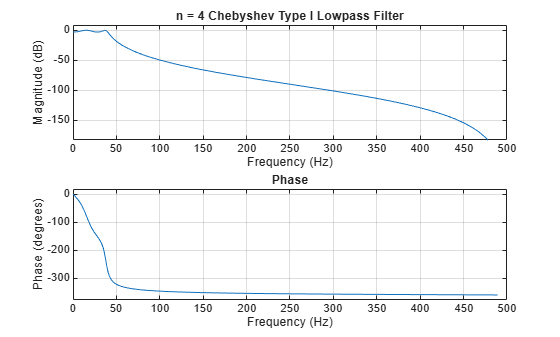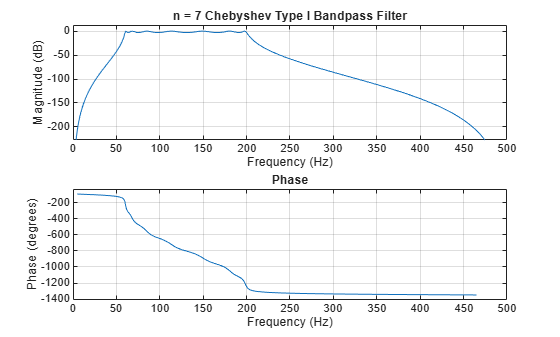# cheb1ord

Chebyshev Type I filter order

## Syntax

``[n,Wp] = cheb1ord(Wp,Ws,Rp,Rs) ``
``[n,Wp] = cheb1ord(Wp,Ws,Rp,Rs,'s') ``

## Description

example

````[n,Wp] = cheb1ord(Wp,Ws,Rp,Rs) `returns the lowest order `n` of the Chebyshev Type I filter that loses no more than `Rp` dB in the passband and has at least `Rs` dB of attenuation in the stopband. The scalar (or vector) of corresponding cutoff frequencies `Wp` is also returned.```
````[n,Wp] = cheb1ord(Wp,Ws,Rp,Rs,'s') `designs a lowpass, highpass, bandpass, or bandstop analog Chebyshev Type I filter with cutoff angular frequencies `Wp`.```

## Examples

collapse all

For data sampled at 1000 Hz, design a lowpass filter with less than 3 dB of ripple in the passband defined from 0 to 40 Hz and at least 60 dB of ripple in the stopband defined from 150 Hz to the Nyquist frequency.

```Wp = 40/500; Ws = 150/500; Rp = 3; Rs = 60; [n,Wp] = cheb1ord(Wp,Ws,Rp,Rs)```
```n = 4 ```
```Wp = 0.0800 ```
```[b,a] = cheby1(n,Rp,Wp); freqz(b,a,512,1000) title('n = 4 Chebyshev Type I Lowpass Filter')```Design a bandpass filter with a passband of 60 Hz to 200 Hz, with less than 3 dB of ripple in the passband and 40 dB attenuation in the stopbands that are 50 Hz wide on both sides of the passband.

```Wp = [60 200]/500; Ws = [50 250]/500; Rp = 3; Rs = 40; [n,Wp] = cheb1ord(Wp,Ws,Rp,Rs)```
```n = 7 ```
```Wp = 1×2 0.1200 0.4000 ```
```[b,a] = cheby1(n,Rp,Wp); freqz(b,a,512,1000) title('n = 7 Chebyshev Type I Bandpass Filter')```## Input Arguments

collapse all

Passband corner (cutoff) frequency, specified as a scalar or a two-element vector with values between 0 and 1 inclusive, with 1 corresponding to the normalized Nyquist frequency, π rad/sample. For digital filters, the unit of passband corner frequency is in radians per sample. For analog filters, passband corner frequency is in radians per second, and the passband can be infinite. The values of `Wp` and `Ws` determine the type of filter cheb1ord returns:

• If `Wp` and `Ws` are both scalars and `Wp` < `Ws`, then `cheb1ord` returns the order and cutoff frequency of a lowpass filter. The stopband of the filter ranges from `Ws` to 1, and the passband ranges from 0 to `Wp`.

• If `Wp` and `Ws` are both scalars and `Wp` > `Ws`, then `cheb1ord` returns the order and cutoff frequency of a highpass filter. The stopband of the filter ranges from 0 to `Ws`, and the passband ranges from `Wp` to 1.

• If `Wp` and `Ws` are both vectors and the interval specified by `Ws` contains the interval specified by `Wp` (`Ws(1)` < `Wp(1)` < `Wp(2)` < `Ws(2)`), then `cheb1ord` returns the order and cutoff frequencies of a bandpass filter. The stopband of the filter ranges from 0 to `Ws(1)` and from `Ws(2)` to 1. The passband ranges from `Wp(1)` to `Wp(2)`.

• If `Wp` and `Ws` are both vectors and the interval specified by `Wp` contains the interval specified by `Ws` (`Wp(1)` < `Ws(1)` < `Ws(2)` < `Wp(2)`), then `cheb1ord` returns the order and cutoff frequencies of a bandstop filter. The stopband of the filter ranges from `Ws(1)` to `Ws(2)`. The passband ranges from 0 to `Wp(1)` and from `Wp(2)` to 1.

Use the following guide to specify filters of different types.

Filter Type Stopband and Passband Specifications

Filter Type

Stopband and Passband Conditions

Stopband

Passband

Lowpass

`Wp` < `Ws`, both scalars

`(Ws,1)`

`(0,Wp)`

Highpass

`Wp` > `Ws`, both scalars

`(0,Ws)`

`(Wp,1)`

Bandpass

The interval specified by `Ws` contains the one specified by `Wp` (```Ws(1) < Wp(1) < Wp(2) < Ws(2)```).

`(0,Ws(1))` and `(Ws(2),1)`

`(Wp(1),Wp(2))`

Bandstop

The interval specified by `Wp` contains the one specified by `Ws` (```Wp(1) < Ws(1) < Ws(2) < Wp(2)```).

`(0,Wp(1))` and `(Wp(2),1)`

`(Ws(1),Ws(2))`

Data Types: `single` | `double`

Note

If your filter specifications call for a bandpass or bandstop filter with unequal ripples in each of the passbands or stopbands, design separate lowpass and highpass filters and cascade the two filters together.

Stopband corner frequency, specified as a scalar or a two-element vector with values between 0 and 1 inclusive, with 1 corresponding to the normalized Nyquist frequency.

• For digital filters, stopband corner frequency is in radians per sample.

• For analog filters, stopband corner frequency is in radians per second and the stopband can be infinite.

Note

The values of `Wp` and `Ws` determine the filter type.

Passband ripple, specified as a scalar in dB.

Data Types: `single` | `double`

Stopband attenuation, specified as a scalar in dB.

Data Types: `single` | `double`

## Output Arguments

collapse all

Lowest filter order, returned as an integer scalar.

Passband corner frequency, returned as a scalar or a two-element vector. Use the output arguments `n` and `Wp` with the `cheby1` function.

## Algorithms

`cheb1ord` uses the Chebyshev lowpass filter order prediction formula described in . The function performs its calculations in the analog domain for both analog and digital cases. For the digital case, it converts the frequency parameters to the s-domain before the order and natural frequency estimation process, and then converts them back to the z-domain.

`cheb1ord` initially develops a lowpass filter prototype by transforming the passband frequencies of the desired filter to 1 rad/s (for lowpass or highpass filters) or to -1 and 1 rad/s (for bandpass or bandstop filters). It then computes the order and natural frequency required for a lowpass filter to match the passband specification exactly when using the values in the `cheby1` function.

 Rabiner, Lawrence R., and Bernard Gold. Theory and Application of Digital Signal Processing. Englewood Cliffs, NJ: Prentice-Hall, 1975.